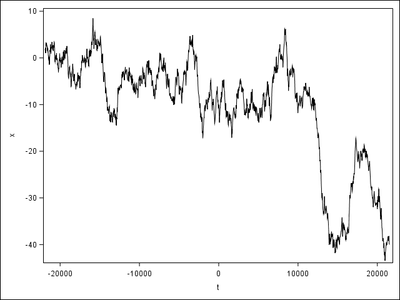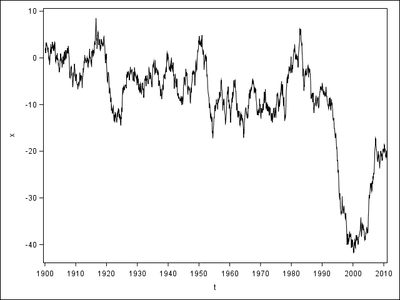## Why Does SGPLOT Trim Some Observations Automatically?

When I apply VALUES and VALUESFORMAT in XAXIS, SGPLOT automatically trims some trailing observations. The left uses a naked format so displays correctly, but the right uses YEAR10 in VALUES and YEAR4. in VALUESFORMAT so ends much earlier than the left. How can I avoid this problem?Here's the code.

``````data have;
t=mdy(1,1,1900);
do until(year(t)>2018);
x+rannor(1);
output;
t=intnx("month",t,1);
end;
run;
ods listing gpath="!userprofile\desktop\";
ods graphics/reset imagename="out";
proc sgplot;
series x=t y=x;
run;
proc sgplot;
series x=t y=x;
xaxis values=("1jan1900"d to "1jan2019"d by year10) valuesformat=year4.;
run;
quit;``````

1 ACCEPTED SOLUTION

Accepted SolutionsDanH_sas
SAS Super FREQ

## Re: Why Does SGPLOT Trim Some Observations Automatically?

Try adding the VALUESHINT option to the XAXIS. This should make the VALUES option place the ticks correctly without constraining the axis range.

Hope this helps!
Dan

3 REPLIES 3

## Re: Why Does SGPLOT Trim Some Observations Automatically?

Your interval is uneven, what happens if it ends at 2020, not 2019 as indicated in your specification?

`xaxis values=("1jan1900"d to "1jan2019"d by year10) valuesformat=year4.;`

My guess is because it's not a full interval it cuts off at the last 10 interval, instead of going past as the default behaviour.

From the documentation:

If the specified range is not evenly divisible by the increment value, the highest value displayed on the axis is the last incremental value below the ending value for the range. For example, this value list produces a maximum axis value of 9:

``values=(0 to 10 by 3)``

@Junyong wrote:

When I apply VALUES and VALUESFORMAT in XAXIS, SGPLOT automatically trims some trailing observations. The left uses a naked format so displays correctly, but the right uses YEAR10 in VALUES and YEAR4. in VALUESFORMAT so ends much earlier than the left. How can I avoid this problem?Here's the code.

``````data have;
t=mdy(1,1,1900);
do until(year(t)>2018);
x+rannor(1);
output;
t=intnx("month",t,1);
end;
run;
ods listing gpath="!userprofile\desktop\";
ods graphics/reset imagename="out";
proc sgplot;
series x=t y=x;
run;
proc sgplot;
series x=t y=x;
xaxis values=("1jan1900"d to "1jan2019"d by year10) valuesformat=year4.;
run;
quit;``````DanH_sas
SAS Super FREQ

## Re: Why Does SGPLOT Trim Some Observations Automatically?

Try adding the VALUESHINT option to the XAXIS. This should make the VALUES option place the ticks correctly without constraining the axis range.

Hope this helps!
Dan

## Re: Why Does SGPLOT Trim Some Observations Automatically?

Any values list will "trim the data" if the limits are less than the range of the values.

It is actually a useful feature as you can quickly modify the amount of data displayed as needed.

Such as

```proc sgplot;
series x=t y=x;
xaxis values=(-1750 -600 0 380 1800);
run;
```

Discussion stats
• 3 replies
• 370 views
• 4 likes
• 4 in conversation